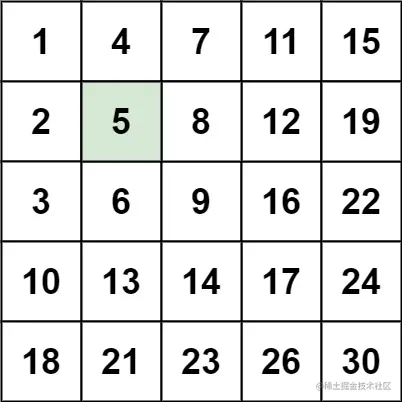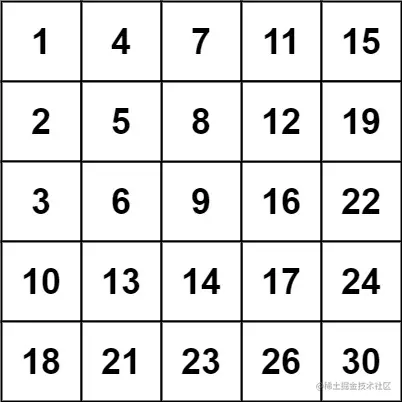# Front end algorithm must brush question series 

2021-08-27 00:15:43

This is my participation 8 The fourth of the yuegengwen challenge 18 God , Check out the activity details ：8 Yuegengwen challenge

There's nothing in this series , It's pure leetcode Topic analysis , Don't seek to use a coquettish line or niche solution , But with clear code and simple enough ideas to help you clear up the topic . Let you no longer be afraid of the written test in the interview .

## 160. Search for a two-dimensional matrix II (search-a-2d-matrix-ii)

• secondary

### subject

leetcode Portal

Write an efficient algorithm to search  m x n  matrix matrix One of the goals in target . The matrix has the following characteristics ：

The elements of each row are arranged in ascending order from left to right . The elements of each column are arranged in ascending order from top to bottom .

Example 1`````` Input ：matrix = [
[1,4,7,11,15],
[2,5,8,12,19],
[3,6,9,16,22],
[10,13,14,17,24],
[18,21,23,26,30]], target = 5
Output ：true
Copy code ``````

Example 2`````` Input ：matrix = [
[1,4,7,11,15],
[2,5,8,12,19],
[3,6,9,16,22],
[10,13,14,17,24],
[18,21,23,26,30]], target = 20
Output ：false
Copy code ``````

### The basic idea

• The idea is actually very clear , It's like walking in a grid
• We can start at the bottom left , In this case 18 The number of
• Bigger than it , Take a step to the right , Smaller than it, take a step up until you find the answer

### The writing method realizes

``````const searchMatrix = function(matrix, target) {
if (matrix.length === 0 || matrix?.length === 0) {
return false
}
//  Boundary value calculation
let rows = matrix.length, cols = matrix?.length
//  Initialize the lower left corner as the starting point ,  The first column of the last row
let row = matrix.length - 1, col = 0
//  You can't go beyond the boundary
while (row >= 0 && col < cols) {
if (matrix[row][col] === target) {
return true
} else if (matrix[row][col] < target) {
//  turn right
col++
} else {
//  Go up
row--
}
}
//  Beyond the boundary
return false
}

let matrix = [
[1,4,7,11,15],
[2,5,8,12,19],
[3,6,9,16,22],
[10,13,14,17,24],
[18,21,23,26,30]], target = 22

console.log(searchMatrix(matrix, target))
Copy code ``````

## 161. Complete square (perfect-squares)

• secondary
• mathematics

### subject

leetcode Portal

Given a positive integer  n, Find some perfect squares （ such as  1, 4, 9, 16, ...） Make their sum equal to n. You need to minimize the number of complete squares that make up the sum .

Give you an integer n , Return and for n Of the complete square of Minimum quantity .

Complete square It's an integer , Its value is equal to the square of another integer ; let me put it another way , Its value is equal to the product of the self multiplication of an integer . for example ,1、4、9 and 16 They're all perfect squares , and 3 and 11 No .

Example 1

`````` Input ： n = 12
Output ： 3
explain ： 12 = 4 + 4 + 4
Copy code ``````

Example 2

`````` Input ： n = 13
Output ： 2
explain ： 13 = 4 + 9
Copy code ``````

### The basic idea

It's still our basic dynamic programming 3 Step train of thought .

#### State definition

our ` The goal is ` Is to seek With `n` The number is ` and ` Of The least number of complete squares

Then set `dp[i]` That is to say i The number is the minimum number of the complete square of the sum , that dp[n] That's our answer to this question

#### State shift

Let's start another j from the beginning , see dp[i - j * j] Actually Namely dp[i] Plus j The square of , So add... To the quantity `1`, The equation is

`dp[i] = Math.min(dp[i], dp[i - j * j] + 1)`

Does anyone say this will not happen , such as `12` Count to `12 = 9 + 3` => `dp = dp + 1`

`12 = 4 + 4 + 4` => `dp = dp + 1 = dp + 1 + 1 = 1 + 1 + 1`

We need to pay attention `dp = 1` , `dp = 3` So when we calculate the minimum , j The minimum value can still be calculated after the change .

#### The border

dp[i] The greatest satisfaction is actually all ` Split into 1 Add up `, `i = 1 + 1 + 1+ ... + 1` (i individual 1)

### The writing method realizes

``````const numSquares = function (n) {
let dp = new Array(n + 1).fill(0)
for (let i = 1; i < n + 1; i++) {
//  This is a  i  Maximum case  i = 1 + 1 + 1+ ... i  individual  1
dp[i] = i
for (let j = 1; i >= j * j; j++) {
//  Follow again  dp[i - j * j] + 1  The biggest one   At present  j  Round up the next smallest bit
dp[i] = Math.min(dp[i], dp[i - j * j] + 1)
}
}
return dp[n]
}

let n = 12
console.log(numSquares(n))
Copy code ``````

In addition, I would like to recommend this series of articles to you , It's very simple , It is very useful for advanced students , Wall crack recommendation ！！！ Core concepts and algorithms

That's all for today , If you want to brush the topic with me, you can add my wechat Click here to make a friend Or Search my wechat `infinity_9368`, You can chat and say Add my code " Heavenly King Gedi tiger " The next sentence is ` english `, Please send me the verification message `presious tower shock the rever monster`, I see it through , After that, I'll do my best to help you , But pay attention to the way you ask questions , I suggest you read this article first ： The wisdom of asking questions